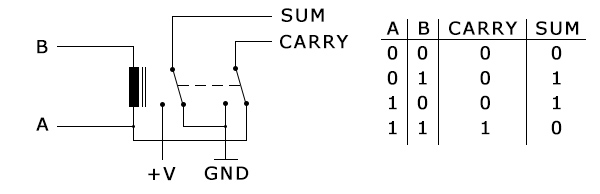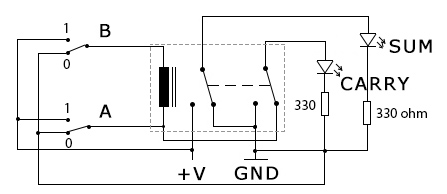## 1bit half adder with one relay

With just one double relay, you can make an adder capable of performing 1bit additions:

0 + 0 = 0
0 + 1 = 1
1 + 0 = 1
1 + 1 = 0 ( with Carry = 1 )The circuit design isn’t mine, I found it with a quick google search.This is the complete schematic of the above circuit.

1.written by: model k on April 22, 2014 at 1:29 am

i don’t understand

2.written by: model k on April 22, 2014 at 1:38 am

that schematic is not clear to me i have a short of seven years of studying electronics as an hobby and i can’t get what is drawn

•written by: Mihai on April 22, 2014 at 9:39 pm

That is a double relay, A and B are inputs ( and as it is shown in the truth table, A and B can only have two values, “1” (V+) or “0” (GND), this is done by switches ), SUM and CARRY are outputs, therefore connected to some indicators ( light bulbs, LED, voltmeters, etc).
V+ is the operating voltage of the relay used.

3.written by: Bilal on May 28, 2014 at 10:07 pm

I wonder if you could make a detailed schematics for your design , I have been trying to make my own copy but I could not manage to work B input , except that all functions are working.

4.written by: akismet on June 28, 2021 at 6:17 am

•written by: Mihai on June 29, 2021 at 2:58 pm